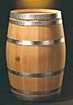Search IntMath
Close

450+ Math Lessons written by Math Professors and Teachers

5 Million+ Students Helped Each Year

1200+ Articles Written by Math Educators and Enthusiasts

Simplifying and Teaching Math for Over 23 Years

# Calculus - an Introduction

By M. Bourne

Calculus is concerned with comparing quantities which vary in a non-linear way. It is used extensively in science and engineering since many of the things we are studying (like velocity, acceleration, current in a circuit) do not behave in a simple, linear fashion. If quantities are continually changing, we need calculus to study what is going on.

## Founders of CalculusSir Isaac Newton
1642-1727Gottfried Leibniz
1646-1716

Calculus was developed independently by the Englishman, Sir Isaac Newton, and by the German, Gottfried Leibniz. They were both working on problems of motion towards the end of the 17th century. There was a bitter dispute between the men over who developed calculus first.

Because of this independent development, we have an unfortunate mix of notation and vocabulary that is used in calculus. From Leibniz we get the dy/dx and int signs, which you will come across later.

The development of an accurate clock in the 17th century led to significant developments in science and mathematics, and amongst the greatest of these was the calculus.

For scientists, it was very important to be able to predict the positions of the stars, to help in maritime navigation. The greatest challenge was to determine longitude when a ship was at sea. Whichever nation could send ships to the New World and successfully bring them back laden with goods, would become a rich country.

## Early application of calculusThe volume of wine barrels was one of the problems solved using the techniques of calculus. See a solution at Volumes by Integration.

Newton and Leibniz built on the algebraic and geometric work of Rene Descartes, who developed the Cartesian co-ordinate system, which we met before.

There are two main branches of calculus.

The first is differentiation (or derivatives), which helps us to find a rate of change of one quantity compared to another.

The second is integration, which is the reverse of differentiation. We may be given a rate of change and we need to work backwards to find the original relationship (or equation) between the two quantities.

## Interactive Applet: Calculus Concepts by First Principles

See some of the basic ideas of calculus by exploring this interactive applet:

Calculus Concepts by First Principles

Continues below

## Calculus in Action 1Solar Two sustainable
energy project in California.

A power tower produces electricity from sunlight by focusing thousands of sun-tracking mirrors, called heliostats, on a single receiver sitting on top of a tower. The receiver captures the thermal energy of the sun and stores it in tanks of molten salt (to the right of the tower) at temperatures greater than 500 degrees centigrade.

When electricity is needed, the energy in the molten salt is used to create steam, which drives a conventional electricity-generating turbine (to the left of the tower).

Calculus (in this case, differentiation) is used to maximise the efficiency of the process.

## Calculus in Action 2Calculus is used to improve the efficiency of hard drives and other computer components.

Now let's move on to the calculus chapters.

## Differentiation

The 3 sections on differentiation in Interactive Mathematics are as follows:

## Integration

The 3 sections on integration in Interactive Mathematics are as follows:

Don't miss the Higher Calculus section which contains more advanced techniques, and very interesting applications.

## Vietnamese translation

Go here for a Vietnamese translation of IntMath calculus.

Array (  => Array ( [dir_ID] => 0 [dir] => calculus [page_url] => calculus-intro.php [page_title] => Calculus - An Introduction [page_ord] => 0 [int_type] => [mob] => staymob [promo_img] => [promo_alt] => )  => Array ( [dir_ID] => 0 [dir] => calculus [page_url] => calculus-concepts-first-principles.php [page_title] => Calculus Concepts First Principles Applet [page_ord] => 30 [int_type] => inter [mob] => staymob [promo_img] => /blog/wp-content/images/2017/11/calculus-first-principles.png [promo_alt] => Calculus First Principles applet )  => Array ( [dir_ID] => 0 [dir] => calculus [page_url] => calculus-vietnamese-translation.php [page_title] => Vietnamese Translation of IntMath Calculus [page_ord] => 50 [int_type] => [mob] => staymob [promo_img] => [promo_alt] => ) )

## Problem SolverThis tool combines the power of mathematical computation engine that excels at solving mathematical formulas with the power of GPT large language models to parse and generate natural language. This creates math problem solver thats more accurate than ChatGPT, more flexible than a calculator, and faster answers than a human tutor. Learn More.